# How to Combine Cells with Different Formats into One Cell with Keeping Format in Excel

Sometimes we need to combine texts in different cells into one cell. It is easy if we use =A1&B1 to combine cells, and we can get proper result if cells have the same cell formatting. But if different formatted numbers, dates or times exist in cells, like content1 in cell A1 is Text, content2 in cell B1 is Date, above formula doesn’t work. If we want to resolve this problem, we can read this article, it will help you to combine cells with different formatting into one cell and at the same time keep their own format properly. It provides two ways to choose, the first one is to strengthen above formula with adding TEXT function, another one is by using Microsoft Word.

## Method 1: Combine Cells with Keeping Cell Formatting by Formula

First, see some examples below.

Example 1: A1-Text & B1-Date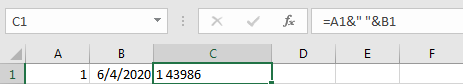Obviously, formatted date value is changed after combining the two cells. And we cannot change it to previous date in format settings.

Example 2: A2-Text & B2-Number (Percentage)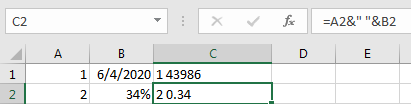We can see that value keeps but format is changed. Above all, we need to strengthen the formula by add Text function, details please see below steps.

Step 1: In C1, enter the formula =A1&” “&TEXT(B1,”m/d/yyyy”). Text function can change date, number format to text, so it can display original value in combined cell. For Text function, there are two parameters, one is value, another one is format text, for date, you can also enter mm/dd/yyyy, dddd to display original value.

Step 2: Click Enter and check final result.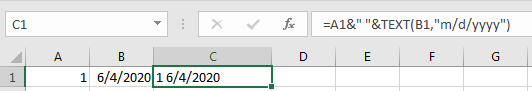Step 3: In C2, enter the formula =A2&” “&TEXT(B2,”0%”). You just need to type number format into Text function to keep B1 original data formatting.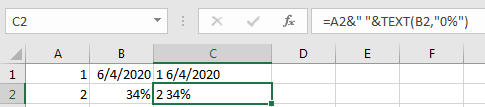Comment:

You can enter Text Function in Help to check more text formats online.

## Method 2: Combine Cells with Keeping Cell Formatting by Microsoft Word

For applying the first method, you need to know the data formatting for all cells. If you don’t know the data formatting, you can choose method 2 to combine cells, see below steps for more details.

Step 1: Copy table from excel to word. Launch word, and create a blank document, then paste copied table on it.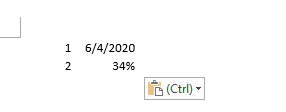Step 2: Select the table on word document. This is important, otherwise Layout tab below is not displayed.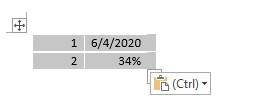Step 3: Click Layout tab in Table Tools. There are two “Layout” tabs, make sure you select the proper one.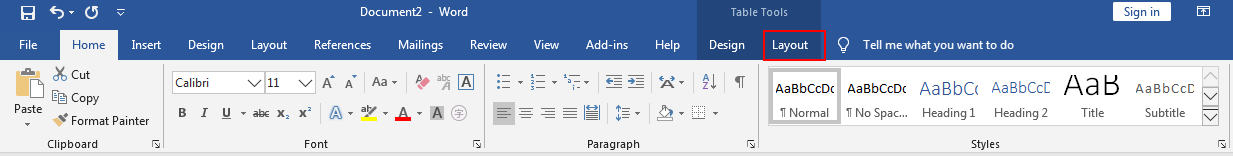Step 4: Click Layout->Convert to Text in Data group.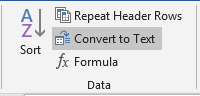Step 5: Select Commas on Convert Table to Text. Then click OK.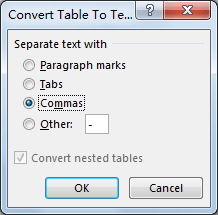Verify that comma is added between two values. And you may find that texts are combined into one cell.Step 6: Copy above table and paste it back to excel worksheet.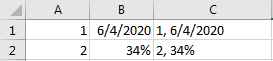Step 7 (Optional): If you want to remove comma between two data, you can use replace function. Press Ctrl+H to pops up Find and Replace dialog. Enter comma into Find what, space into Replace with, then click Replace All.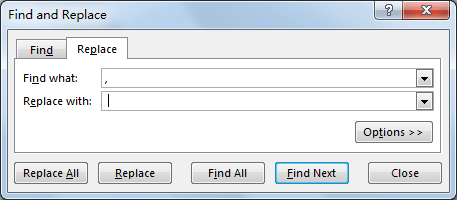Verify that comma is removed.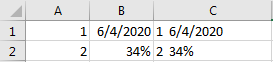Comment:

In step 5, if you select Tab as text separator, the two data is still displayed into two cells after pasting it back to excel.

Related Posts

Calculate Years Between Dates In Ms Excel

If you are an avid Ms Excel user, then you might have come across a task in which you needed to calculate the years between the dates; you might take it easy and do this task manually, which is also ...

Calculate Number of Hours between Two Times

Calculating the difference between two times might be a valuable statistic for subsequent computations or averages, whether you're producing a time sheet for staff or recording personal exercises. While Excel has a plethora of complex functions, including date and time ...

Calculate Loan Interest in Given Year

When you borrow money, you are supposed to repay it gradually. Lenders, on the other hand, want to be compensated for their services and the risk they incur by lending you money. That is, you will not just repay the ...

Calculate Interest Rate for Loan

The interest rate is the fee charged by a lender to a borrower and is expressed as a percentage of the principal—the lent amount. The interest rate on a loan is often expressed as an annual percentage rate, abbreviated as ...

Calculate Interest for Given Period

Using the IPMT function in Excel, we can compute the interest payment on any loan. This step-by-step tutorial will guide Excel users of all skill levels through the process to calculate interest for given period. Finally, the formula: =IPMT(B3/12,1,B5,-B2) The ...

How To Use Excel GCD Function

This post will guide you how to use Excel GCD function with syntax and examples in Microsoft excel. Description The Excel GCD function Returns the greatest common divisor of two or more integers. So you can use the GCD function ...

Calculate A Ratio From Two Numbers In Excel

In elementary mathematics, a ratio is a connection or comparison between two or more integers. For example, ratios are often expressed as ":" to demonstrate the relationship between two numbers. You would think that manually calculating a ratio from two ...

How To Use Excel RRI Function

This post will guide you how to use Excel RRI function with syntax and examples in Microsoft excel. Description The Excel RRI function Returns an equivalent interest rate for the growth of an investment. So you can use the RRI ...

CAGR Formula Examples in Excel

CAGR in Excel is a formula that calculates the compound annual growth rate for any invested amount over the specified years or timeframe. Although there is no direct function in Excel that can help us identify the CAGR value, there ...

Build Hyperlink With VLOOKUP in Excel

You might have come across a task in which you were assigned to build hyperlinks, which seems very easy, and if you are new to excel or don't have enough experience with it, then you might wonder about doing this ...

Sidebar